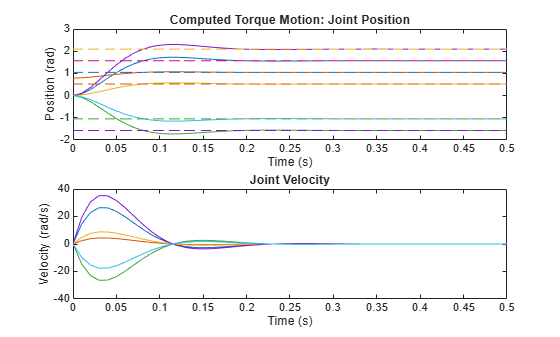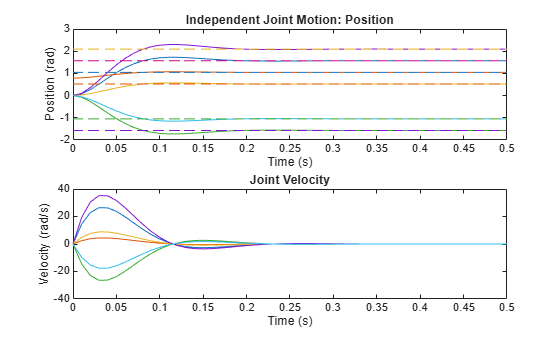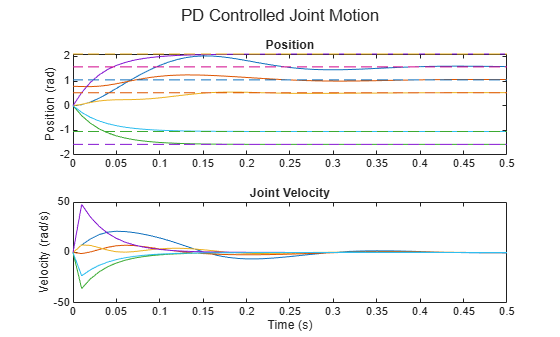Simulate Joint-Space Trajectory Tracking in MATLAB

This example shows how to simulate the joint-space motion of a robotic manipulator under closed-loop control.

Define Robot and Initial State

Load an ABB IRB-120T from the robot library using the loadrobot function.

numJoints = numel(homeConfiguration(robot));

Define simulation parameters, including the time range over which the trajectory is simulated, the initial state as [joint configuration; jointVelocity], and the joint-space set point.

% Set up simulation parameters
tSpan = 0:0.01:0.5;
q0 = zeros(numJoints,1);
q0(2) = pi/4; % Something off center
qd0 = zeros(numJoints,1);
initialState = [q0; qd0];

% Set up joint control targets
targetJointPosition = [pi/2 pi/3 pi/6 2*pi/3 -pi/2 -pi/3]';
targetJointVelocity = zeros(numJoints,1);
targetJointAcceleration = zeros(numJoints,1);

Visualize the goal position.

show(robot,targetJointPosition)ans =
Axes (Primary) with properties:

XLim: [-1 1]
YLim: [-1 1]
XScale: 'linear'
YScale: 'linear'
GridLineStyle: '-'
Position: [0.1300 0.1100 0.7750 0.8150]
Units: 'normalized'

Show all properties

Model Behavior with Joint-Space Control

Using a jointSpaceMotionModel object, simulate the closed-loop motion of the model under a variety of controllers. This example compares a few of them. Each instance uses the derivative function to compute the state derivative. Here, the state is 2n-element vector [joint configuration; joint velocity], where n is the number of joints in the associated rigidBodyTree object.

Computed-Torque Control

Computed-torque control uses an inverse-dynamics computation to compensate for the robot dynamics. The controller drives the closed-loop error dynamics of each joint based on a second-order response.

Create a jointSpaceMotionModel and specify the robot model. Set the "MotionType" to "ComputedTorqueControl". Update the error dynamics using updateErrorDynamicsFromStep and specify the desired settling time and overshoot respectively. Alternatively, you can set the damping ratio and natural frequency directly in the object.

computedTorqueMotion = jointSpaceMotionModel("RigidBodyTree",robot,"MotionType","ComputedTorqueControl");
updateErrorDynamicsFromStep(computedTorqueMotion,0.2,0.1);

This motion model requires position, velocity, and acceleration to be provided.

qDesComputedTorque = [targetJointPosition; targetJointVelocity; targetJointAcceleration];

To view an example of this controller in practice in Simulink, see the Perform Safe Trajectory Tracking Control Using Robotics Manipulator Blocks example.

Independent Joint Control

With independent joint control, model each joint as a separate system that has a second-order tracking response. This type of model is an idealized behavior, and is best used when the response is slow, or when the dynamics will not have a significant impact on the resultant trajectory. In those cases, it will behave the same as computed-torque control, but with less computational overhead.

Create another joinSpaceMotionModel using the "IndependentJointMotion" motion type.

IndepJointMotion = jointSpaceMotionModel("RigidBodyTree",robot,"MotionType","IndependentJointMotion");
updateErrorDynamicsFromStep(IndepJointMotion,0.2,0.1);

This motion model requires position, velocity, and acceleration to be provided.

qDesIndepJoint = [targetJointPosition; targetJointVelocity; targetJointAcceleration];

Proportional-Derivative Control

Proportional-Derivative Control, or PD Control, combines gravity compensation with proportional and derivative gains. Despite the simpler nature relative to other closed-form models, the PD Controller can be stable for all positive gain values, which makes it a desirable option. Here, the PD Gains are set as n-by-n matrices, where n is the number of joints in the associated rigidBodyTree object. For this robot, n = 6. Additionally, PD Control does not require an acceleration profile, so its state vector is just a 2n-element vector of joint configurations and joint velocities.

pdMotion = jointSpaceMotionModel("RigidBodyTree",robot,"MotionType","PDControl");
pdMotion.Kp = diag(300*ones(1,6));
pdMotion.Kd = diag(10*ones(1,6));

This motion model requires position and velocity to be provided.

qDesPD = [targetJointPosition; targetJointVelocity];

Simulate using an ODE Solver

The derivative function outputs the state derivative, which can be integrated using an ordinary differential equation (ODE) solver such as ode45. For each motion model, the ODE solver outputs a m-element column vector that covers tspan and a 2-by-m matrix of the 2n-element state vector at each instant in time.

Calculate the trajectory for each motion model, using the most appropriate ODE solver for each system.

[tComputedTorque,yComputedTorque] = ode45(@(t,y)derivative(computedTorqueMotion,y,qDesComputedTorque),tSpan,initialState);
[tIndepJoint,yIndepJoint] = ode45(@(t,y)derivative(IndepJointMotion,y,qDesIndepJoint),tSpan,initialState);
[tPD,yPD] = ode15s(@(t,y)derivative(pdMotion,y,qDesPD),tSpan,initialState);

Plot Results

Once the simulation is complete, compare the results side-by-side. Each plot shows the joint position on the top, and velocity on the bottom. The dashed lines indicate the reference trajectories, while the solid lines display the simulated response.

% Computed Torque Control
figure
subplot(2,1,1)
plot(tComputedTorque,yComputedTorque(:,1:numJoints)) % Joint position
hold all
plot(tComputedTorque,targetJointPosition*ones(1,length(tComputedTorque)),'--') % Joint setpoint
title('Computed Torque Motion: Joint Position')
xlabel('Time (s)')
subplot(2,1,2)
plot(tComputedTorque,yComputedTorque(:,numJoints+1:end)) % Joint velocity
title('Joint Velocity')
xlabel('Time (s)')In the following plot, use independent joint control to confirm that the computed torque motion behaves equivalently under some simplifying assumptions.

% Independent Joint Motion
figure
subplot(2,1,1)
plot(tIndepJoint,yIndepJoint(:,1:numJoints))
hold all
plot(tIndepJoint,targetJointPosition*ones(1,length(tIndepJoint)),'--')
title('Independent Joint Motion: Position')
xlabel('Time (s)')
subplot(2,1,2);
plot(tIndepJoint,yIndepJoint(:,numJoints+1:end))
title('Joint Velocity')
xlabel('Time (s)')Finally, the PD Controller uses fairly aggressive gains to achieve similar rise times, but unlike the other approaches, the individual joints behave differently, since each joint and the associated bodies have slightly different dynamic properties that are not compensated by the controller.

% PD with Gravity Compensation
figure
subplot(2,1,1)
plot(tPD,yPD(:,1:numJoints))
hold all
plot(tPD,targetJointPosition*ones(1,length(tPD)),'--')
title('PD Controlled Joint Motion: Position')
xlabel('Time (s)')
subplot(2,1,2)
plot(tPD,yPD(:,numJoints+1:end))
title('Joint Velocity')
xlabel('Time (s)')Visualize the Trajectories as an Animation

To see what this behavior looks like in 3-D, the following example helper plots the robot motion in time. The third input is the number of frames between each sample.

exampleHelperRigidBodyTreeAnimation(robot,yComputedTorque,1);exampleHelperRigidBodyTreeAnimation(robot,yIndepJoint,1);exampleHelperRigidBodyTreeAnimation(robot,yPD,1);